# Line Plots and Stacked/Clustered Bar Plots¶

Tyler Caraza-Harter

Previously, we learned how to create matplotlib pie charts, simple bar graphs, and scatter plots (by calling Pandas plotting methods for Series and DataFrames).

In this document, we'll also learn how to create line plots and more complicated bar plots (clustered and stacked).

Let's start by doing our matplotlib setup and usual imports:

In :
%matplotlib inline

In :
import pandas as pd
from pandas import Series, DataFrame


For readability, you may also want to increase the default font size at the start of your notebooks. You can do so by copy/pasting the following:

In :
import matplotlib
matplotlib.rcParams.update({'font.size': 16})


# Line Plot from a Series¶

We can create a line plot from either a Series (with s.plot.line()) or a DataFrame (with df.plot.line()).

In :
s = Series([0,100,300,200,400])
s

Out:
0      0
1    100
2    300
3    200
4    400
dtype: int64
In :
s.plot.line()

Out:
<matplotlib.axes._subplots.AxesSubplot at 0x113e047b8>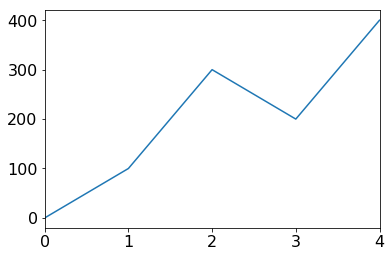The y values are clearly the values in the Series, but where are the x-values coming from? You guessed it, the Series' index. Let's try the same values with a different index.

In :
s = Series([0,100,300,200,400], index=[1,2,30,31,32])
s

Out:
1       0
2     100
30    300
31    200
32    400
dtype: int64
In :
s.plot.line()

Out:
<matplotlib.axes._subplots.AxesSubplot at 0x115eb1c88>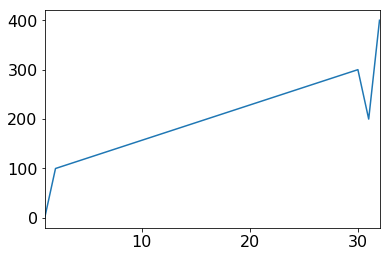Now we see that the plot starts from 1 (instead of 0) and a bigger gap in the index (between 2 and 30) corresponds to a bigger line segment over the x-axis.

What happens if our index is not in order?

In :
s = Series([0,100,300,200,400], index=[1,11,2,22,3])
s

Out:
1       0
11    100
2     300
22    200
3     400
dtype: int64
In :
s.plot.line()

Out:
<matplotlib.axes._subplots.AxesSubplot at 0x11600c978>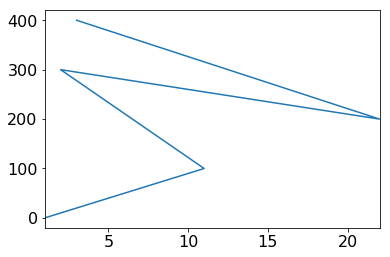Oops! That's probably not what we want. 99% of the time, people making a line plot want readers to be able to lookup a single y-value (per line) given a point along the x-axis. So even though this line passes through all of our data points, the lines between the points are very misleading.

If your data isn't already sorted, you'll probably want to sort it by the index first:

In :
s.sort_index()

Out:
1       0
2     300
3     400
11    100
22    200
dtype: int64

Don't get confused about this function! If we have a Python list L and we call L.sort(), the items in L are rearranged in place and the sort function doesn't return anything.

In contrast, if we have a Pandas Series s and we call s.sort_index(), the items in s are not moved, but the sort_index function returns a new Series that is sorted. So if we print s again, we see the original (unsorted) data:

In :
s

Out:
1       0
11    100
2     300
22    200
3     400
dtype: int64

Because sort_index() returns a new Series and we can call .plot.line() on a Series, we can do the following on an unsorted Series s in one step:

In :
s.sort_index().plot.line()

Out:
<matplotlib.axes._subplots.AxesSubplot at 0x1160e93c8>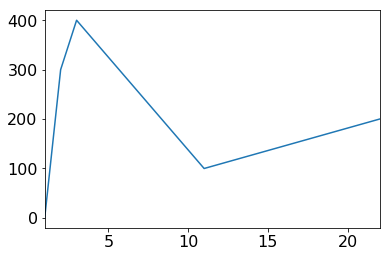# Line Plot from a DataFrame¶

In addition to the Series.plot.line() method, there is also a DataFrame.plot.line() method. Whereas the line function for a Series creates a plot with a single line, the line plot for a DataFrame draws a line for each column in the DataFrame (remember that each column in a DataFrame is essentially just a Series).

Let's try with a DataFrame containing temperature patterns for Madison, WI. The data was copied from https://www.usclimatedata.com/climate/madison/wisconsin/united-states/uswi0411, and contains the typical daily highs and lows for each month of the year.

In :
df = DataFrame({
"high": [26, 31, 43, 57, 68, 78, 82, 79, 72, 59, 44, 30],
"low": [11, 15, 25, 36, 46, 56, 61, 59, 50, 39, 28, 16]
})

df

Out:
high low
0 26 11
1 31 15
2 43 25
3 57 36
4 68 46
5 78 56
6 82 61
7 79 59
8 72 50
9 59 39
10 44 28
11 30 16
In :
df.plot.line()

Out:
<matplotlib.axes._subplots.AxesSubplot at 0x1161bce80>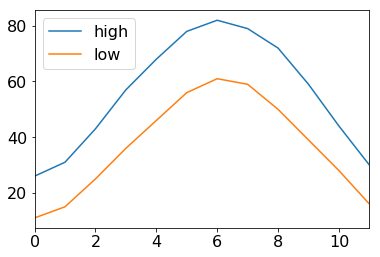Not bad! We can see the temperatures vary througout the year, with highs correlated with lows. But what is the x-axis? What is the y-axis?

Remember that calling an AxesSubplot object. There are AxesSubplot.set_xlabel and AxesSubplot.set_ylabel functions that will help us out here. Just to make sure to call them in the same cell where .plot.line is called, or the plot will be displayed before they can have an effect.

In :
ax = df.plot.line()
ax.set_xlabel('Month')
ax.set_ylabel('Temp (Fehrenheit)')

Out:
Text(0, 0.5, 'Temp (Fehrenheit)')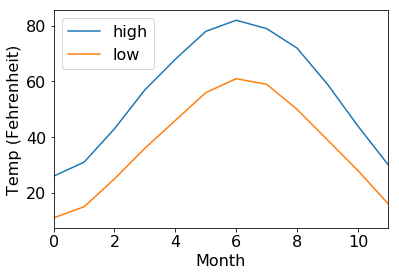What if we want the plot in Celcius? That's easy enough with some element-wise operations.

In :
c_df = (df - 32) * 5/9
c_df

Out:
high low
0 -3.333333 -11.666667
1 -0.555556 -9.444444
2 6.111111 -3.888889
3 13.888889 2.222222
4 20.000000 7.777778
5 25.555556 13.333333
6 27.777778 16.111111
7 26.111111 15.000000
8 22.222222 10.000000
9 15.000000 3.888889
10 6.666667 -2.222222
11 -1.111111 -8.888889
In :
ax = c_df.plot.line()
ax.set_xlabel('Month')
ax.set_ylabel('Temp (Celsius)')

Out:
Text(0, 0.5, 'Temp (Celsius)')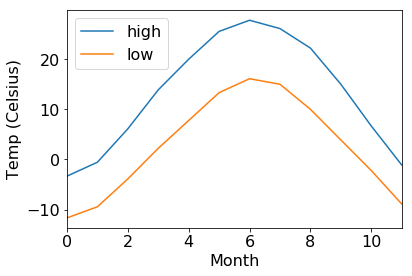That's looking good!

One small thing: did you notice the extra print above the plot that says Text(0,0.5,'Temp (Celsius)')? That happened because the call to set_ylabel returned that value. We could always put None at the end of our cell to supress that:

In :
ax = c_df.plot.line()
ax.set_xlabel('Month')
ax.set_ylabel('Temp (Celsius)')
None## Tick Labels¶

The above plot would be nicer if we saw actual month names along the y-axis. Let's create a DataFrame with the same data, but month names for the index.

In :
df = DataFrame({
"month": ["Jan", "Feb", "Mar", "Apr", "May", "Jun", "Jul", "Aug", "Sep", "Oct", "Nov", "Dec"],
"high": [26, 31, 43, 57, 68, 78, 82, 79, 72, 59, 44, 30],
"low": [11, 15, 25, 36, 46, 56, 61, 59, 50, 39, 28, 16]
})

df = df.set_index("month")


Out:
high low
month
Jan 26 11
Feb 31 15
Mar 43 25
Apr 57 36
May 68 46

Let's try plotting it.

In :
ax = df.plot.line()
ax.set_xlabel('Month')
ax.set_ylabel('Temp (Fehrenheit)')
None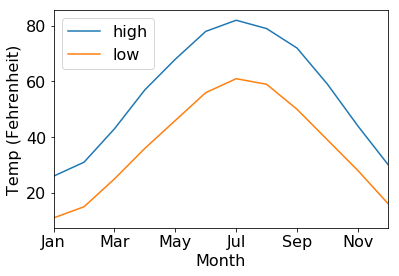Unfortunately, even though we now have months for the index, matplotlib won't use them for the x-axis unless we specifically tell it to. We can explicitly give matplotlib tick labels with the set_xticklabels method.

In :
# careful, this is an example of a bad plot!
ax = df.plot.line()
ax.set_xticklabels(df.index)
None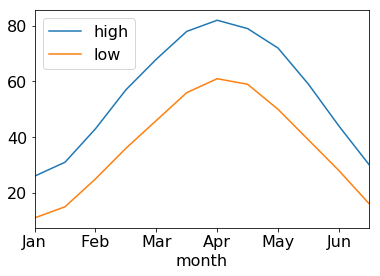Yikes! That's not what we wanted at all. The above plot starts at Feb (instead of Jan), and it only covers half a year. We've set the tick labels, but not the tick positions. Let's take a look at the positions:

In :
ax.get_xticks()

Out:
array([ 0.,  2.,  4.,  6.,  8., 10., 12.])

You should read the above as follows:

• the first tick label (Jan) is drawn at position -2, which is out of the plots range (so we don't see Jan)
• the second tick label (Feb) is drawn at position 0 (the leftmost)
• the third tick label (Mar) is drawn at position 2
• and so on

Fortunately, we can set the tick positions explicitly. The only correct configuration in this case is 0, 1, 2, 3, ...

In :
ax = df.plot.line()
ax.set_xticks([0, 1, 2, 3])
ax.set_xticklabels(df.index)
None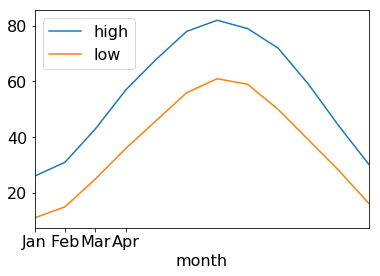If we want to count from 0 to 11, we can use range(len(df.index)).

In :
ax = df.plot.line()
ax.set_xticks(range(len(df.index)))
ax.set_xticklabels(df.index)
None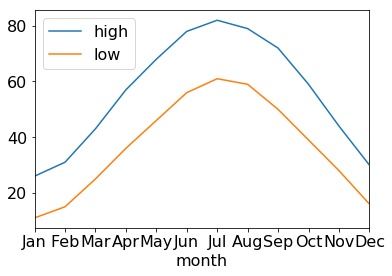This plot is correct, but crowded! There are two solutions: (1) make the plot wider or (2) rotate the labels. We'll demo both. We'll also add back the axis labels.

In :
# approach 1: wider plot
ax = df.plot.line(figsize=(8,4)) # this is the (width,height)
ax.set_xticks(range(len(df.index)))
ax.set_xticklabels(df.index)
ax.set_xlabel('Month')
ax.set_ylabel('Temp (Fehrenheit)')
None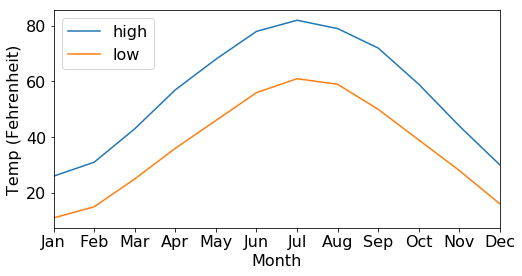In :
# approach 2: rotate ticks
ax = df.plot.line()
ax.set_xticks(range(len(df.index)))
ax.set_xticklabels(df.index, rotation=90) # 90 is in degrees
ax.set_xlabel('Month')
ax.set_ylabel('Temp (Fehrenheit)')
None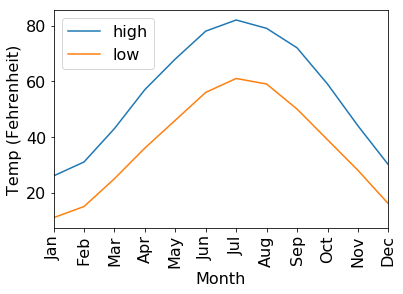# Example: Stock Market Returns¶

In this example, we'll plot the performance of American stocks from 1970 to 2017. Specifically, we'll be looking at S&P 500 index data. The S&P 500 index tracks how well the 500 largest public American companies are collectively worth (think of it as a weighted average with more valuable companies being weighted more heavily).

We'll get our data from the Wikipedia on the S&P 500 Index article. Take a moment to skip the article.

We're interested in the "Total Annual Return Including Dividends" column of the table in the "Annual returns" section. Investors make money when (1) stock prices rise, or (2) companies pay dividends to shareholders. This column captures the yearly return, considering both these factors.

There are three parts in this example. In part 1, we do some web scraping to collect the data (it's a details BeautifulSoup example). For part 2, we'll visualise the data in several ways. In part 3, we'll simulate stock market returns, sampling from the real data in order to explore possible investment outcomes.

## Stock Market Part 1: Collecting the Data¶

As a first step, let's download the wiki page and save it to a file named sp500.html. We check if this file exists before doing the download. If it does, we just use the contents of sp500.html instead of fetching the data again from Wikipedia (it's faster to access data on your computer rather than from a website).

In :
import os, requests

path = "sp500.html"

if not os.path.exists(path):
r = requests.get('https://en.wikipedia.org/wiki/S%26P_500_Index')
r.raise_for_status()
f = open(path, "w")
f.write(r.text)
f.close()

f = open(path)
f.close()

In :
# let's parse the HTML
from bs4 import BeautifulSoup
page = BeautifulSoup(html, 'html.parser')


The page contains six tables. Which one has the data we care about? We can loop over each table, convert the contents to text, and check with the text contains the term "Total Annual Return" (that's the name of the column with the data we want).

In :
target_column = "Total Annual Return"
tab = None
for curr in page.find_all('table'):
if curr.get_text().find(target_column) >= 0:
tab = curr
break
assert(tab != None)


Now we have the table we want. Let's create a list of lists representing the table data. This will be a list of rows, where each row contains td (table data) and th (table header) elements. Both of these elements are used to represent cells in HTML tables.

In :
rows = []
for tr in tab.find_all('tr'):
rows.append(tr.find_all(['td', 'th']))

# let's print the first three rows to make sure they are what we expect.
rows[:3]

Out:
[[<th>Year
</th>, <th>Change in Index
</th>, <th>Total Annual Return Including Dividends
</th>, <th>Value of 1.00 Invested on 1970‑01‑01 </th>, <th>5 Year Annualized Return </th>, <th>10 Year Annualized Return </th>, <th>15 Year Annualized Return </th>, <th>20 Year Annualized Return </th>, <th>25 Year Annualized Return </th>], [<td>1970 </td>, <td align="right">0.10% </td>, <td align="right">4.01% </td>, <td align="right">1.04
</td>, <td align="right">-
</td>, <td align="right">-
</td>, <td align="right">-
</td>, <td align="right">-
</td>, <td align="right">-
</td>], [<td>1971
</td>, <td align="right">10.79%
</td>, <td align="right">14.31%
</td>, <td align="right">1.19 </td>, <td align="right">- </td>, <td align="right">- </td>, <td align="right">- </td>, <td align="right">- </td>, <td align="right">- </td>]] Let's make sure (with asserts) that the 0th and 2nd columns contain year and annual return data. If they do, we want to extract these entries and construct a Series with year as index and annual return for values. In : assert(rows.get_text().find("Year") >= 0) assert(rows.get_text().find("Total Annual Return") >= 0) index = [] values = [] for row in rows[1:]: index.append(row.get_text().strip()) values.append(row.get_text().strip()) if index[-1] == '2018': break returns = Series(values, index=index) returns.tail()  Out: 2014 13.69% 2015 1.38% 2016 11.96% 2017 21.83% 2018 −4.43% dtype: object Let's normalize the data so we can use it to multiply initial money. For example, we want to convert 4% to 1.04. That way, if we start with \100, we can multiply by 1.04 to compute that we have \\$104 after a year. Don't worry about the replace of chr(8722). It's not important to the example. In : print("'{}' is a weird dash, not the negative dash '-' that will let us convert to a float.".format(chr(8722))) mults = returns.str.replace(chr(8722), "-").str.replace("%", "").astype(float) / 100 + 1 mults.head()  '−' is a weird dash, not the negative dash '-' that will let us convert to a float.  Out: 1970 1.0401 1971 1.1431 1972 1.1898 1973 0.8534 1974 0.7353 dtype: float64 We'll save this nicely formatted data to a CSV file. Any analysis of returns can use that directly without needing to repeat this HTML parsing. In : df = DataFrame({"year":mults.index, "return":mults.values}) df.to_csv("sp500.csv", index=False) df.tail()  Out: year return 44 2014 1.1369 45 2015 1.0138 46 2016 1.1196 47 2017 1.2183 48 2018 0.9557 ## Stock Market Part 2: Plotting¶ In the previous step, we generated sp500.csv. Let's read that in and start doing some plotting. There are a few things we want to plot: • returns each year • total returns over time • correlation between the returns in one year and the subsequent year In : df = pd.read_csv("sp500.csv") df.tail()  Out: year return 44 2014 1.1369 45 2015 1.0138 46 2016 1.1196 47 2017 1.2183 48 2018 0.9557 Lets use the year as the index. In : df = df.set_index("year") df.head()  Out: return year 1970 1.0401 1971 1.1431 1972 1.1898 1973 0.8534 1974 0.7353 Plot 1: returns each year. We want the year for the x-axis and the return on the y-axis. In : df.plot.line()  Out: <matplotlib.axes._subplots.AxesSubplot at 0x117520cc0>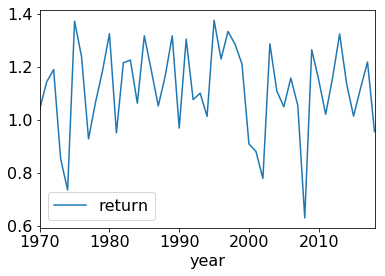We see a lot of noise, but the line stays above 1 in most years. Plot 2: total returns over time. The x-axis will be time, and the y-axis will be total returns. We will assume we started in 1970 with \$1000.

In order to get the total money in a given year, we want to multiply the starting money by all the return multiples up through that year (this is called a compounding return). We can use the cumprod method for this.

In :
df['return'].cumprod().head()

Out:
year
1970    1.040100
1971    1.188938
1972    1.414599
1973    1.207219
1974    0.887668
Name: return, dtype: float64

For example, the 1973 value of 1.207 came by multiplying 1.0401 * 1.1431 * 1.1898 * 0.8534 (the multiples for 1970 through 1973). Let's plot how much money we have over time, if we start with $1000. In : total = 1000 * df['return'].cumprod() total.head()  Out: year 1970 1040.100000 1971 1188.938310 1972 1414.598801 1973 1207.218617 1974 887.667849 Name: return, dtype: float64 In : ax = total.plot.line() ax.set_ylabel('Net Worth') None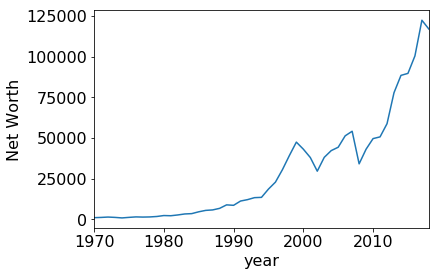Plot 3: do a scatter to show the correlation between one year and the next. To do this, we'll create two Series, both indexed by year. The first Series we'll pull directly from sp500.csv: the index will be a year, and the corresponding value will be the returns for that year. In the second Series, the index will be a year, and the value will the the returns in the year FOLLOWING the year in the index. In : df = pd.read_csv("sp500.csv") df.head()  Out: year return 0 1970 1.0401 1 1971 1.1431 2 1972 1.1898 3 1973 0.8534 4 1974 0.7353 In : df = df.set_index("year") df.head()  Out: return year 1970 1.0401 1971 1.1431 1972 1.1898 1973 0.8534 1974 0.7353 In : series1 = df['return'] series2 = Series(df['return'].values[1:], index=df['return'].index[:-1]) pairs = DataFrame({"curr":series1, "next":series2}) pairs.head()  Out: curr next year 1970 1.0401 1.1431 1971 1.1431 1.1898 1972 1.1898 0.8534 1973 0.8534 0.7353 1974 0.7353 1.3720 As you can see, the next column of the 1970 year contains the curr value of the 1971 year. Let's do a scatter plot to look at the correlation. As a pre-step, we'll subtract 1 from ever cell so a 10% loss will be represented as -0.1 (instead of 0.9). In : (pairs - 1).head()  Out: curr next year 1970 0.0401 0.1431 1971 0.1431 0.1898 1972 0.1898 -0.1466 1973 -0.1466 -0.2647 1974 -0.2647 0.3720 In : (pairs - 1).plot.scatter(x='curr', y='next')  Out: <matplotlib.axes._subplots.AxesSubplot at 0x117665ac8>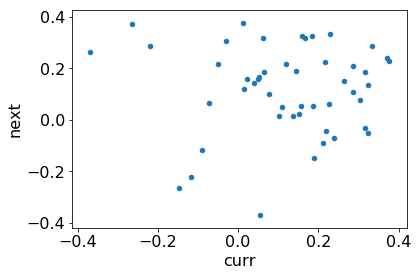## Stock Market Part 3: Simulation¶ In this section, we'll going explore likely outcomes if one were to invest \$1000 in an S&P 500 index fund for 10 years.

In :
df = pd.read_csv("sp500.csv")

Out:
year return
0 1970 1.0401
1 1971 1.1431
2 1972 1.1898
3 1973 0.8534
4 1974 0.7353
In :
returns = df['return']

Out:
0    1.0401
1    1.1431
2    1.1898
3    0.8534
4    0.7353
Name: return, dtype: float64
In :
import random
sim = DataFrame()

# do 25 simulations
for i in range(25):
# sample returns for 10 years

# start with $1000, compute compounded wealth over # the course of the decade net_worth = 1000 * Series(decade).cumprod() # add this simulation as a column in the DataFrame sim['sim'+str(i)] = net_worth sim  Out: sim0 sim1 sim2 sim3 sim4 sim5 sim6 sim7 sim8 sim9 ... sim15 sim16 sim17 sim18 sim19 sim20 sim21 sim22 sim23 sim24 0 1285.800000 1184.400000 1189.800000 735.300000 1062.700000 1160.000000 1375.800000 1065.600000 1054.900000 1333.600000 ... 1065.600000 779.000000 969.000000 1229.600000 1304.700000 779.000000 1166.100000 1143.100000 1229.600000 1186.700000 1 1702.270620 1303.787520 1081.528200 745.005960 669.501000 1345.600000 1212.217380 1079.665920 1161.233920 1739.947920 ... 909.383040 1025.865100 1245.940200 1691.683680 1521.410670 686.376900 1027.450710 1086.859480 1405.555760 1374.079930 2 2086.302872 1185.142856 1229.589411 834.108673 846.650965 1419.473440 1606.188028 1323.238552 1207.799400 1915.334670 ... 1186.472052 1351.372096 1156.481694 2320.990009 1412.173384 722.411687 1353.460820 1169.678172 1094.927937 1306.475197 3 1983.656771 1247.362856 1310.250476 1104.276472 938.766590 1655.247978 1872.975860 1621.761169 1471.462009 2020.486544 ... 1526.752237 1153.260947 1348.573303 2414.061708 889.669232 894.634633 1578.270663 736.897249 1325.300775 1704.558190 4 1803.144004 1577.415067 1362.791520 1270.580509 1080.144838 1678.090400 2267.049981 2086.882272 1746.183966 2339.521369 ... 2010.580021 1372.149875 849.601181 2464.998410 979.347890 830.399867 1827.479600 766.446828 1823.348806 1620.693927 5 2320.285705 1159.873299 1381.598043 1340.335379 1279.323546 1805.960889 1766.031935 2471.703363 2246.989528 2462.346241 ... 2136.643388 1427.173085 535.248744 2594.410827 1053.974200 882.465938 2384.312634 907.779623 2169.420410 1961.687929 6 2352.305648 1376.421644 1472.230875 1659.871333 1349.558409 2408.429442 1881.883630 3254.986159 2889.179135 3212.623140 ... 2369.110189 1217.949510 652.093545 2949.585669 1387.978624 940.355704 2643.725849 825.171678 2199.358411 2230.243007 7 2467.803855 1072.232461 1648.309687 1694.894618 1856.722459 2591.951765 1789.294956 4002.330981 3031.037831 3726.642843 ... 2693.441374 1107.116105 789.294027 3509.417029 1851.008292 1254.058367 3131.228895 788.616572 2546.637104 2717.105055 8 1554.716429 1355.945170 1995.114045 2232.006722 1687.760715 3083.904210 1660.823578 4956.486686 3726.964116 3909.621007 ... 3062.173498 1371.052584 939.102033 4621.551286 2539.583377 1660.247872 4148.878286 1084.978680 2851.214902 3288.783959 9 2048.027951 1410.318571 1757.894985 2122.191992 2234.426411 3452.739154 1748.016816 6138.113113 3180.591177 5180.247834 ... 3940.404857 1806.087569 1112.272448 3398.226660 2922.044634 1975.362918 4206.132807 1144.544010 2890.561668 4330.999596 10 rows × 25 columns Each of the above columns in the above DataFrame represents a simulation. The bottom row represents the total wealth after 10 years. Let's plot each simulation. We'll disable the legend because 25 legend entries is too many. In : sim.set_index(sim.index + 1, inplace=True) ax = sim.plot.line(legend=False, figsize=(8,8)) ax.set_xlabel("Years Elapsed") ax.set_ylabel("Net Worth ($)")

Out:
Text(0, 0.5, 'Net Worth (\$)')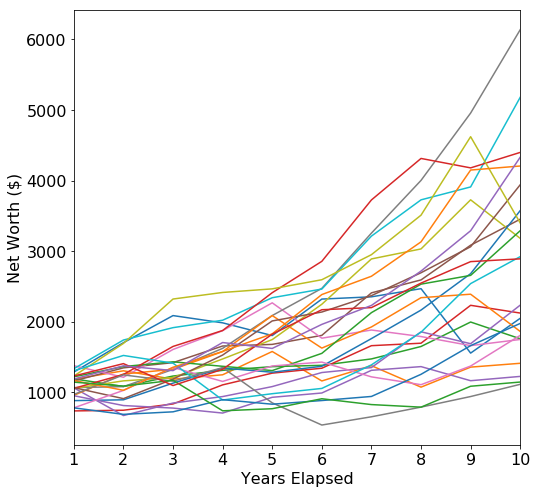It appears that doubling one's money (or better) over 10 years is fairly like. Of course, in some cases wealth increases very little (or worse, decreases). We also observe that the road to wealth is usually bumpy.

# Bar Plots¶

Just like a line plot, bar plots can be created from either a Pandas Series or DataFrame. For our example data, let's learn a bit about the fire hydrants around the city of Madison. Data describing each fire hydrant can be found at http://data-cityofmadison.opendata.arcgis.com/datasets/54c4877f16084409849ebd5385e2ee27_6. We have already downloaded the data to a file named "Fire_Hydrants.csv". Let's read it and preview a few rows.

In :
df = pd.read_csv('Fire_Hydrants.csv')

Out:
X Y OBJECTID CreatedBy CreatedDate LastEditor LastUpdate FacilityID DataSource ProjectNumber ... Elevation Manufacturer Style year_manufactured BarrelDiameter SeatDiameter Comments nozzle_color MaintainedBy InstallType
0 -89.519573 43.049308 2536 NaN NaN WUJAG 2018-06-07T19:45:53.000Z HYDR-2360-2 FASB NaN ... 1138.0 NaN Pacer 1996.0 5.0 NaN NaN blue MADISON WATER UTILITY NaN
1 -89.521988 43.049193 2537 NaN NaN WUJAG 2018-06-07T19:45:53.000Z HYDR-2360-4 FASB NaN ... 1170.0 NaN Pacer 1995.0 5.0 NaN NaN blue MADISON WATER UTILITY NaN
2 -89.522093 43.048233 2538 NaN NaN WUJAG 2018-06-07T19:45:53.000Z HYDR-2361-19 FASB NaN ... 1179.0 NaN Pacer 1996.0 5.0 NaN NaN blue MADISON WATER UTILITY NaN
3 -89.521013 43.049033 2539 NaN NaN WUJAG 2018-06-07T19:45:53.000Z HYDR-2360-3 FASB NaN ... 1163.0 NaN Pacer 1995.0 5.0 NaN NaN blue MADISON WATER UTILITY NaN
4 -89.524782 43.056263 2540 NaN NaN WUPTB 2017-08-31T16:19:46.000Z HYDR-2257-5 NaN NaN ... 1065.0 NaN Pacer 1996.0 5.0 NaN NaN blue MADISON WATER UTILITY NaN

5 rows × 25 columns

For our first example, let's see what nozzle colors are most common. We can get a Series summarizing the data by first extracting the nozzle_color column, then using the Series.value_counts() function to produce a summary Series.

In :
df['nozzle_color'].head()

Out:
0    blue
1    blue
2    blue
3    blue
4    blue
Name: nozzle_color, dtype: object
In :
df['nozzle_color'].value_counts()

Out:
blue      5810
Blue      1148
Green      320
Orange      74
BLUE        45
green        9
Red          9
orange       4
C            1
GREEN        1
white        1
ORANGE       1
Name: nozzle_color, dtype: int64

The above data means, for example, that there are 5810 "blue" nozzles and 1148 "Blue" nozzles. We can already see there is a lot of blue, but we would really like a total count, not confused by whether the letters are upper or lower case.

In :
df['nozzle_color'].str.upper().value_counts()

Out:
BLUE      7003
GREEN      330
ORANGE      79
RED          9
C            1
WHITE        1
Name: nozzle_color, dtype: int64

Great! It's not clear what "C" means, but the data is clean enough. Let's plot it with Series.plot.bar.

In :
counts = df['nozzle_color'].str.upper().value_counts()
ax = counts.plot.bar()
ax.set_ylabel("Hydrant Counts")

Out:
Text(0, 0.5, 'Hydrant Counts')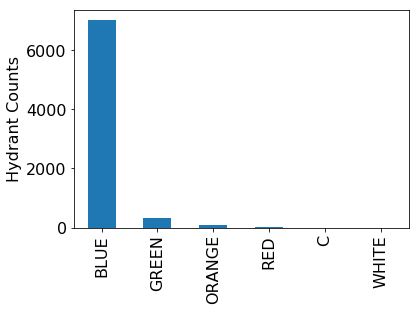Is the data reasonable? Try to notice next time you're walking by a hydrant. Consider it a challenge to spot a green nozzle (bonus points for orange!).

For our second question, let's create a similar plot that tells us what model of hydrants are most common. The model is represented by the Style column in the table. The following code is a copy/paste of above, just replacing "nozzle_color" with "Style":

In :
counts = df['Style'].str.upper().value_counts()
counts.plot.bar(color="gray")

Out:
<matplotlib.axes._subplots.AxesSubplot at 0x117973828>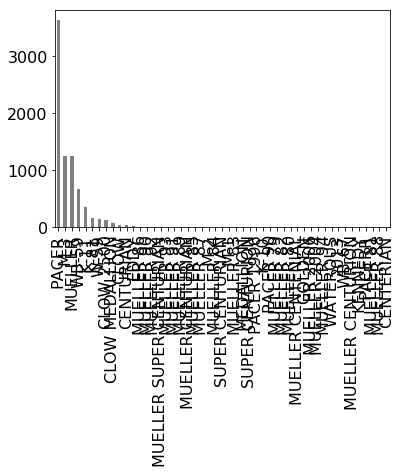Woah! That's way too much data. Let's just consider the top 10 models.

In :
top10 = counts[:10]
top10

Out:
PACER             3620
M-3               1251
MUELLER           1243
WB-59              664
K-11               351
K-81               162
W-59               151
CLOW 2500          123
CLOW MEDALLION      70
CLOW                50
Name: Style, dtype: int64

How many others are not in the top 10? We should show that in our results too.

In :
others = counts[10:].sum()
top10["others"] = others
top10

Out:
PACER             3620
M-3               1251
MUELLER           1243
WB-59              664
K-11               351
K-81               162
W-59               151
CLOW 2500          123
CLOW MEDALLION      70
CLOW                50
others             229
Name: Style, dtype: int64

Now that looks like what we want to plot.

In :
ax = top10.plot.bar(color="gray")
ax.set_ylabel("Hydrant Counts")

Out:
Text(0, 0.5, 'Hydrant Counts')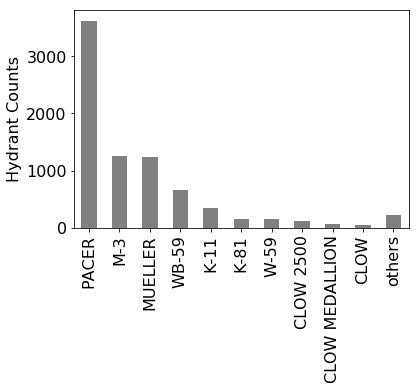Nice! This shows us what we want. We see Pacer is easily the most common. Some of the longer texts are harder to read vertically, so we also have the option to use .barh instead of .bar to rotate the bars.

In :
top10.plot.barh(color="gray")

Out:
<matplotlib.axes._subplots.AxesSubplot at 0x116a0ef28>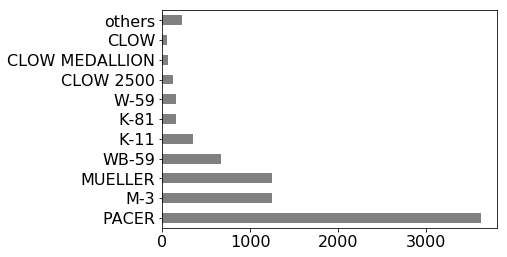I wonder what is up with all those Pacer hydrants? Have they always been so popular with the city? Turns out we can find out, because we also have a column called year_manufactured.

Let's find all the rows for Pacer hydrants and extract the year.

In :
pacer_years = df[df['Style'] == 'Pacer']['year_manufactured']

Out:
0    1996.0
1    1995.0
2    1996.0
3    1995.0
4    1996.0
Name: year_manufactured, dtype: float64

Let's round to the decade. We can do that by dividing by 10 (integer division), then multiplying by 10 again.

In :
pacer_decades = pacer_years // 10 * 10

Out:
0    1990.0
1    1990.0
2    1990.0
3    1990.0
4    1990.0
Name: year_manufactured, dtype: float64

How many Pacers were there each decade?

In :
pacer_decades.value_counts().astype(int)

Out:
2000.0    1730
1990.0     846
2010.0     503
1980.0      21
1960.0       1
Name: year_manufactured, dtype: int64

Let's do the same thing in one step for non-pacers. That is, we'll identify non-pacers, extract the year, round to the decade, and then count how many entries there are per decade.

In :
other_decades = (df[df['Style'] != 'Pacer']['year_manufactured'] // 10 * 10)

Out:
2010.0    1196
1980.0     937
1970.0     578
1990.0     431
1950.0     371
1960.0     349
2000.0     215
1940.0      68
1930.0       9
1900.0       1
Name: year_manufactured, dtype: int64

Let's line up these two Series side-by-side in a DataFrame

In :
pacer_df = DataFrame({
})
pacer_df

Out:
pacer other
1900 NaN 1
1930 NaN 9
1940 NaN 68
1950 NaN 371
1960 1.0 349
1970 NaN 578
1980 21.0 937
1990 846.0 431
2000 1730.0 215
2010 503.0 1196

That looks plottable!

In :
pacer_df.plot.bar()

Out:
<matplotlib.axes._subplots.AxesSubplot at 0x1169ea400>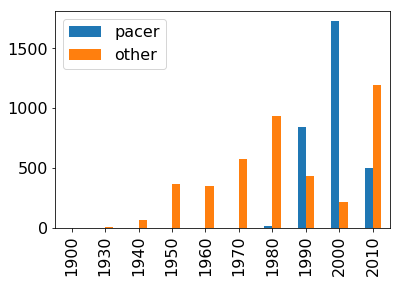That plot shows that the city started getting Pacers in the 90's. Most were from the 2000 decade, and it seems there is finally a shift to other styles.

While this plot is fine, when multiple bars represent a breakdown of a total amount, it's more intuitive to stack the bars over each other. This is easy with the stacked= argument.

In :
ax = pacer_df.plot.bar(stacked=True)
ax.set_ylabel("Hydrant Counts")

Out:
Text(0, 0.5, 'Hydrant Counts')